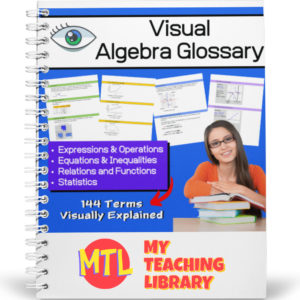Showing all 5 results

•\$1.50

Students will complete a crossword puzzle by answering compound interest word problems. Also included, an optional number search puzzle which will provide an answer bank to use when completing the crossword puzzle! Answer Keys provided.

Sample problem: How much interest does a \$472 investment earn at 7% compounded annually over eight years?

•\$1.50

Students will complete a crossword puzzle by answering simple interest word problems. Also included, an optional number search puzzle which will provide an answer bank to use when completing the crossword puzzle! Answer Keys provided.

Sample problem: If you put \$5.62 in a saving account that pays 3% for eight years, what is the amount of money you will have at the end of the eight years?

•\$3.50

Give students the practice they need when learning to solve one-step, one-variable equations! This resource provides 7 different worksheets, each with 25 problems for a total of 175 problems. All equations in this set of worksheets use only the properties of addition and subtraction.

•\$15.00

Give your Algebra I and Algebra II students a tool they can really use! This Visual Algebra Glossary will be an invaluable resource giving them explanations, definitions and examples of 144 terms. Easy to follow and understand, students will turn to this resource while learning new concepts, completing work and studying for exams.

There are four color coded sections, each with an term index:

• – Expressions & Operations
• – Equations & Inequalities
• – Relations & Functions
• – Statistics

73 pages

•\$4.00

This 123 page math resource will help students who are learning (or struggling to understand) algebra better by giving detailed explanations of related terminology and concepts. This book as been categorized as curriculum because of the detailed explanations of the topics. It however, is for instructional use only and does not include student problems.

(See description below for covered topics)